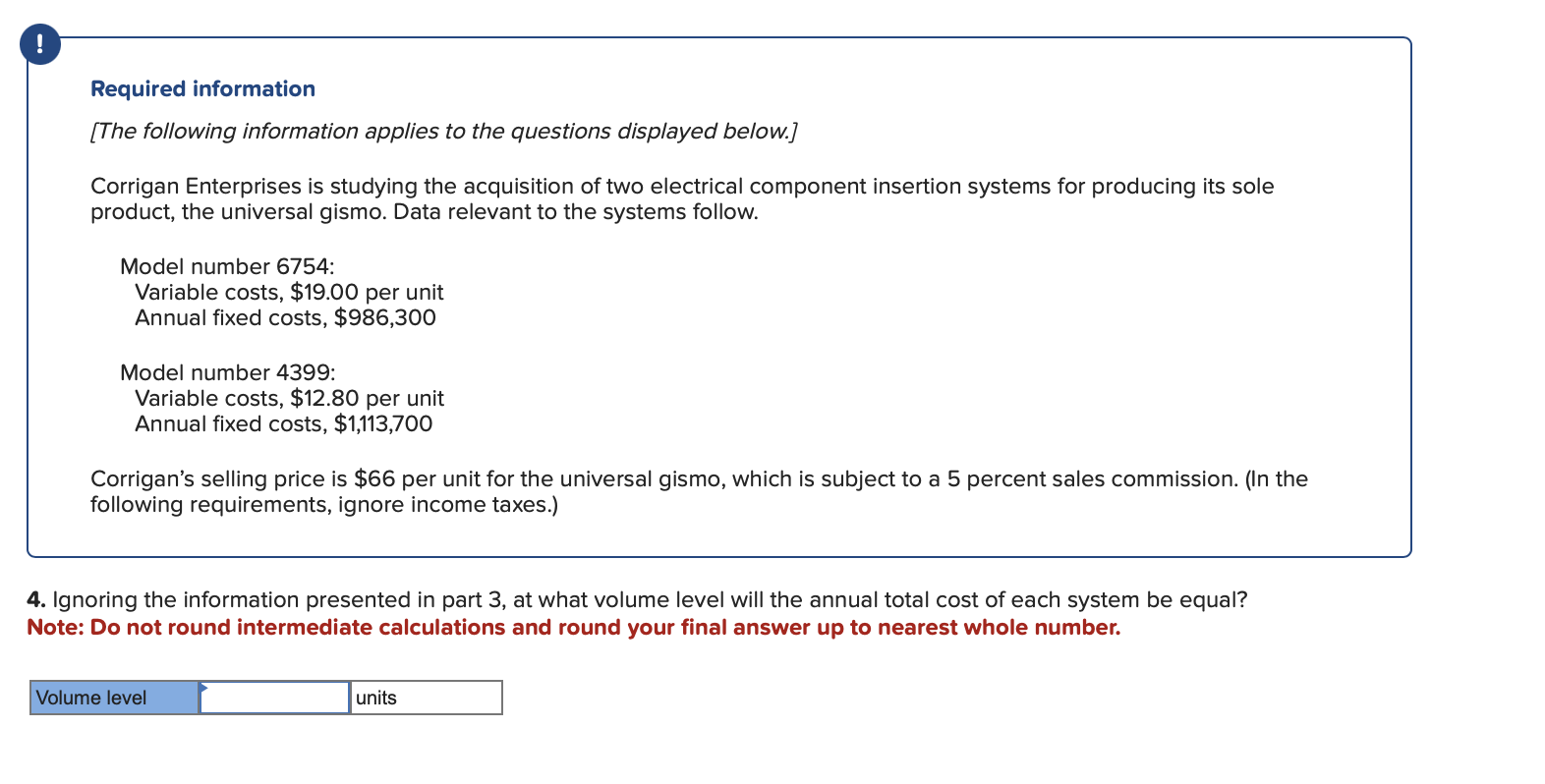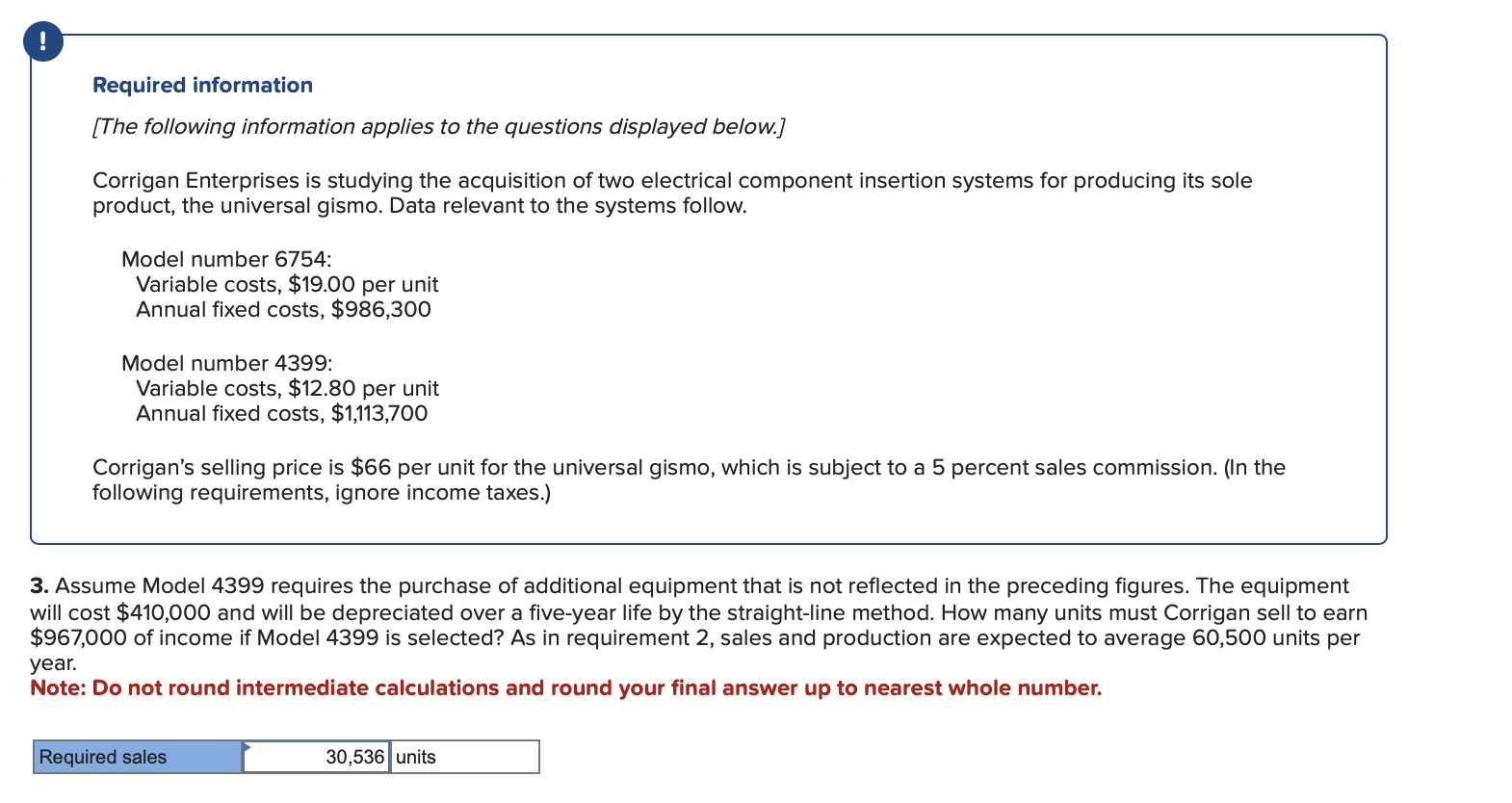# (Solved): I'm stuck on the last two parts of this question. Please advise. Required information [The follow ...I'm stuck on the last two parts of this question. Please advise.

Required information [The following information applies to the questions displayed below.] Corrigan Enterprises is studying the acquisition of two electrical component insertion systems for producing its sole product, the universal gismo. Data relevant to the systems follow. Model number 6754: Variable costs, per unit Annual fixed costs, Model number 4399: Variable costs, per unit Annual fixed costs, Corrigan's selling price is per unit for the universal gismo, which is subject to a 5 percent sales commission. (In the following requirements, ignore income taxes.) 4. Ignoring the information presented in part 3, at what volume level will the annual total cost of each system be equal? Note: Do not round intermediate calculations and round your final answer up to nearest whole number. Required information [The following information applies to the questions displayed below.] Corrigan Enterprises is studying the acquisition of two electrical component insertion systems for producing its sole product, the universal gismo. Data relevant to the systems follow. Model number 6754: Variable costs, per unit Annual fixed costs, Model number 4399: Variable costs, per unit Annual fixed costs, Corrigan's selling price is per unit for the universal gismo, which is subject to a 5 percent sales commission. (In the following requirements, ignore income taxes.) 3. Assume Model 4399 requires the purchase of additional equipment that is not reflected in the preceding figures. The equipment will cost and will be depreciated over a five-year life by the straight-line method. How many units must Corrigan sell to earn of income if Model 4399 is selected? As in requirement 2, sales and production are expected to average 60,500 units per year. Note: Do not round intermediate calculations and round your final answer up to nearest whole number.

We have an Answer from Expert

W.N.1: Calculation of the Total Variable Cost per unit of Both modelsModel 6754=$19+($66×5%)=$22.30Model 4399=$12.80